# Introduction To Prims Algorithm

suggest change

Let’s say we have 8 houses. We want to setup telephone lines between these houses. The edge between the houses represent the cost of setting line between two houses.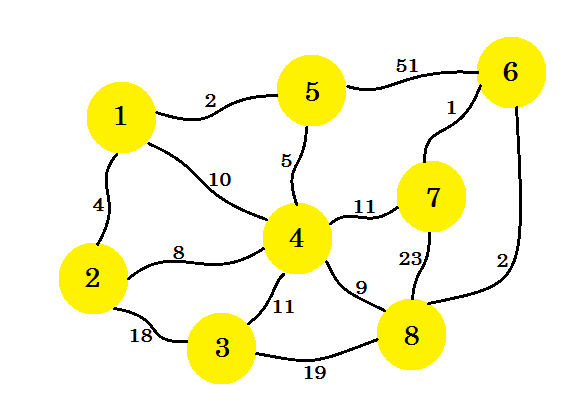Our task is to set up lines in such a way that all the houses are connected and the cost of setting up the whole connection is minimum. Now how do we find that out? We can use Prim’s Algorithm.

Prim’s Algorithm is a greedy algorithm that finds a minimum spanning tree for a weighted undirected graph. This means it finds a subset of the edges that forms a tree that includes every node, where the total weight of all the edges in the tree are minimized. The algorithm was developed in 1930 by Czech mathematician Vojtěch Jarník and later rediscovered and republished by computer scientist Robert Clay Prim in 1957 and Edsger Wybe Dijkstra in 1959. It is also known as DJP algorithm, Jarnik’s algorithm, Prim-Jarnik algorithm or Prim-Dijsktra algorithm.

Now let’s look at the technical terms first. If we create a graph, S using some nodes and edges of an undirected graph G, then S is called a subgraph of the graph G. Now S will be called a Spanning Tree if and only if:

• It contains all the nodes of G.
• It is a tree, that means there is no cycle and all the nodes are connected.
• There are (n-1) edges in the tree, where n is the number of nodes in G.

There can be many Spanning Tree‘s of a graph. The Minimum Spanning Tree of a weighted undirected graph is a tree, such that sum of the weight of the edges is minimum. Now we’ll use Prim’s algorithm to find out the minimum spanning tree, that is how to set up the telephone lines in our example graph in such way that the cost of set up is minimum.

At first we’ll select a source node. Let’s say, node-1 is our source. Now we’ll add the edge from node-1 that has the minimum cost to our subgraph. Here we mark the edges that are in the subgraph using the color blue. Here 1-5 is our desired edge.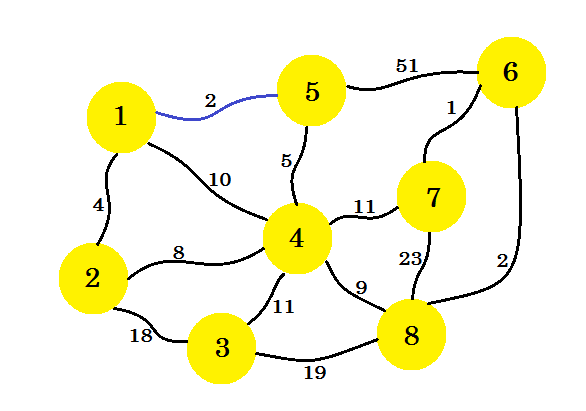Now we consider all the edges from node-1 and node-5 and take the minimum. Since 1-5 is already marked, we take 1-2.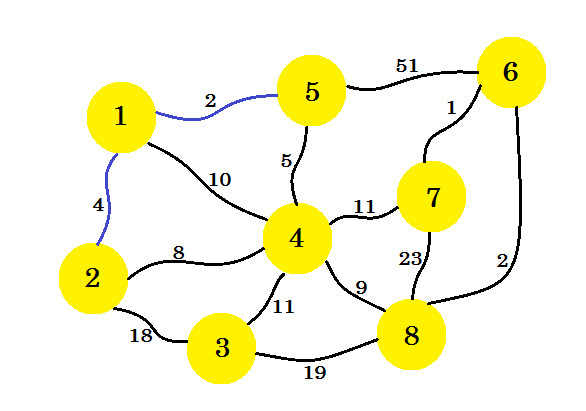This time, we consider node-1, node-2 and node-5 and take the minimum edge which is 5-4.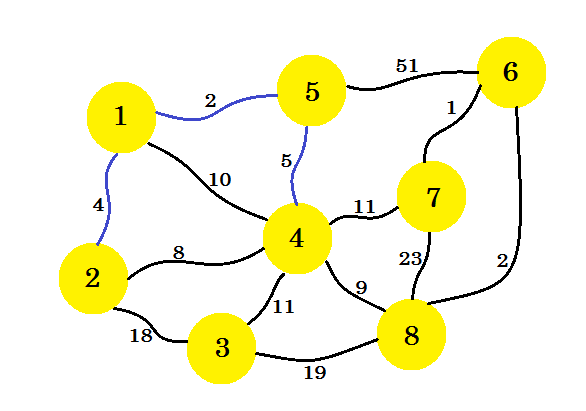The next step is important. From node-1, node-2, node-5 and node-4, the minimum edge is 2-4. But if we select that one, it’ll create a cycle in our subgraph. This is because node-2 and node-4 are already in our subgraph. So taking edge 2-4 doesn’t benefit us. We’ll select the edges in such way that it adds a new node in our subgraph. So we select edge 4-8.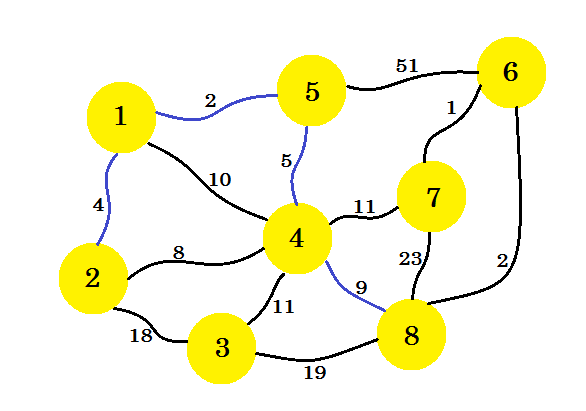If we continue this way, we’ll select edge 8-6, 6-7 and 4-3. Our subgraph will look like: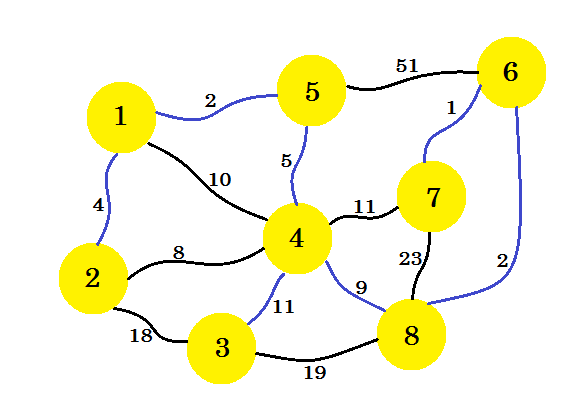This is our desired subgraph, that’ll give us the minimum spanning tree. If we remove the edges that we didn’t select, we’ll get: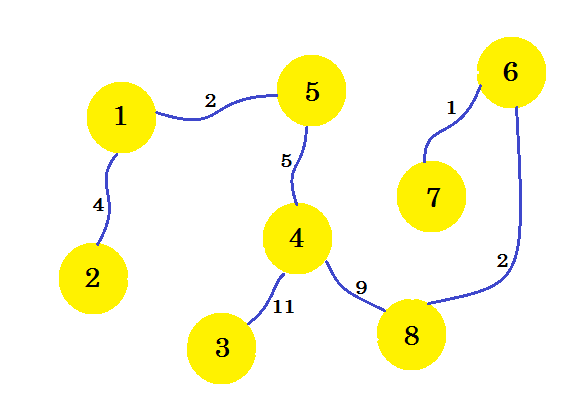This is our minimum spanning tree (MST). So the cost of setting up the telephone connections is: 4 + 2 + 5 + 11 + 9 + 2 + 1 = 34. And the set of houses and their connections are shown in the graph. There can be multiple MST of a graph. It depends on the source node we choose.

The pseudo-code of the algorithm is given below:

``````Procedure PrimsMST(Graph):     // here Graph is a non-empty connected weighted graph
Vnew[] = {x}                   // New subgraph Vnew with source node x
Enew[] = {}
while Vnew is not equal to V
u -> a node from Vnew
v -> a node that is not in Vnew such that edge u-v has the minimum cost
// if two nodes have same weight, pick any of them
add edge (u, v) to Enew
end while
Return Vnew and Enew``````

Complexity:

Time complexity of the above naive approach is O(V²). It uses adjacency matrix. We can reduce the complexity using priority queue. When we add a new node to Vnew, we can add its adjacent edges in the priority queue. Then pop the minimum weighted edge from it. Then the complexity will be: O(ElogE), where E is the number of edges. Again a Binary Heap can be constructed to reduce the complexity to O(ElogV).

The pseudo-code using Priority Queue is given below:

``````Procedure MSTPrim(Graph, source):
for each u in V
key[u] := inf
parent[u] := NULL
end for
key[source] := 0
Q = Priority_Queue()
Q = V
while Q is not empty
u -> Q.pop
for each v adjacent to i
if v belongs to Q and Edge(u,v) < key[v]    // here Edge(u, v) represents
// cost of edge(u, v)
parent[v] := u
key[v] := Edge(u, v)
end if
end for
end while``````

Here key[] stores the minimum cost of traversing node-v. parent[] is used to store the parent node. It is useful for traversing and printing the tree.

Below is a simple program in Java:

``````import java.util.*;

public class Graph
{
private static int infinite = 9999999;
int      NNodes;
Graph(int[][] mat)
{
int i, j;
NNodes = mat.length;
for ( i=0; i < NNodes; i++)
{
for ( j=0; j < NNodes; j++)
{
if ( LinkCost[i][j] == 0 )
}
}
for ( i=0; i < NNodes; i++)
{
for ( j=0; j < NNodes; j++)
if ( LinkCost[i][j] < infinite )
System.out.print( " " + LinkCost[i][j] + " " );
else
System.out.print(" * " );
System.out.println();
}
}
public int unReached(boolean[] r)
{
boolean done = true;
for ( int i = 0; i < r.length; i++ )
if ( r[i] == false )
return i;
return -1;
}
public void Prim( )
{
int i, j, k, x, y;
boolean[] Reached = new boolean[NNodes];
int[] predNode = new int[NNodes];
Reached = true;
for ( k = 1; k < NNodes; k++ )
{
Reached[k] = false;
}
predNode = 0;
printReachSet( Reached );
for (k = 1; k < NNodes; k++)
{
x = y = 0;
for ( i = 0; i < NNodes; i++ )
for ( j = 0; j < NNodes; j++ )
{
if ( Reached[i] && !Reached[j] &&
{
x = i;
y = j;
}
}
System.out.println("Min cost edge: (" +
+ x + "," +
+ y + ")" +
predNode[y] = x;
Reached[y] = true;
printReachSet( Reached );
System.out.println();
}
int[] a= predNode;
for ( i = 0; i < NNodes; i++ )
System.out.println( a[i] + " --> " + i );
}
void printReachSet(boolean[] Reached )
{
System.out.print("ReachSet = ");
for (int i = 0; i < Reached.length; i++ )
if ( Reached[i] )
System.out.print( i + " ");
//System.out.println();
}
public static void main(String[] args)
{
int[][] conn = {{0,3,0,2,0,0,0,0,4},  // 0
{3,0,0,0,0,0,0,4,0},  // 1
{0,0,0,6,0,1,0,2,0},  // 2
{2,0,6,0,1,0,0,0,0},  // 3
{0,0,0,1,0,0,0,0,8},  // 4
{0,0,1,0,0,0,8,0,0},  // 5
{0,0,0,0,0,8,0,0,0},  // 6
{0,4,2,0,0,0,0,0,0},  // 7
{4,0,0,0,8,0,0,0,0}   // 8
};
Graph G = new Graph(conn);
G.Prim();
}
}``````

Compile the above code using `javac Graph.java`

Output:

``````\$ java Graph
*  3  *  2  *  *  *  *  4
3  *  *  *  *  *  *  4  *
*  *  *  6  *  1  *  2  *
2  *  6  *  1  *  *  *  *
*  *  *  1  *  *  *  *  8
*  *  1  *  *  *  8  *  *
*  *  *  *  *  8  *  *  *
*  4  2  *  *  *  *  *  *
4  *  *  *  8  *  *  *  *
ReachSet = 0 Min cost edge: (0,3)cost = 2
ReachSet = 0 3
Min cost edge: (3,4)cost = 1
ReachSet = 0 3 4
Min cost edge: (0,1)cost = 3
ReachSet = 0 1 3 4
Min cost edge: (0,8)cost = 4
ReachSet = 0 1 3 4 8
Min cost edge: (1,7)cost = 4
ReachSet = 0 1 3 4 7 8
Min cost edge: (7,2)cost = 2
ReachSet = 0 1 2 3 4 7 8
Min cost edge: (2,5)cost = 1
ReachSet = 0 1 2 3 4 5 7 8
Min cost edge: (5,6)cost = 8
ReachSet = 0 1 2 3 4 5 6 7 8
0 --> 0
0 --> 1
7 --> 2
0 --> 3
3 --> 4
2 --> 5
5 --> 6
1 --> 7
0 --> 8``````